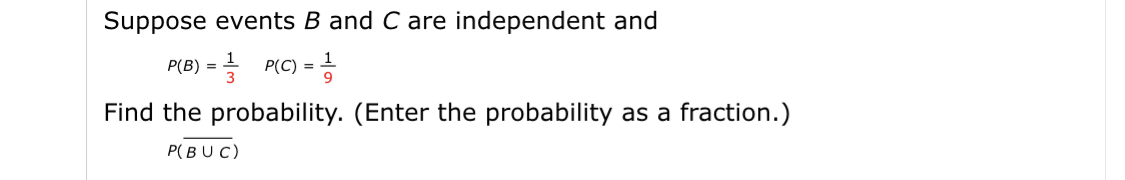# Suppose events B and C are independent andP(B) %=D 글 P(C) =D증Find the probability. (Enter the probability as a fraction.)P(BUC)

Question
3 viewshelp_outlineImage TranscriptioncloseSuppose events B and C are independent and P(B) %=D 글 P(C) =D증 Find the probability. (Enter the probability as a fraction.) P(BUC) fullscreen
check_circle

Step 1

Independent Events:

The events A and B are said to be independent, if the result of one of the events does not depend on the results of the other events. In other words, the events A and B are said to be independent, if the occurrence or non-occurrence of ...

### Want to see the full answer?

See Solution

#### Want to see this answer and more?

Solutions are written by subject experts who are available 24/7. Questions are typically answered within 1 hour.*

See Solution
*Response times may vary by subject and question.
Tagged in

### Other